### Home > INT1 > Chapter Ch11 > Lesson 11.2.3 > Problem11-88

11-88.
1. Copy the graph at right onto graph paper. 11-88 HW eTool (Desmos). Homework Help ✎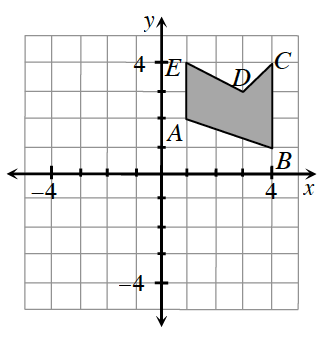1. If the shape ABCDE were rotated about the origin 180°, where would point A′ be?

2. If the shape ABCDE were reflected across the x-axis, where would point C′ be?

3. If the shape were translated so that each point (x, y) corresponds to (x − 1, y + 3), where would point B′ be?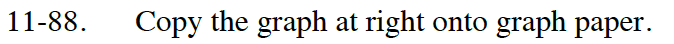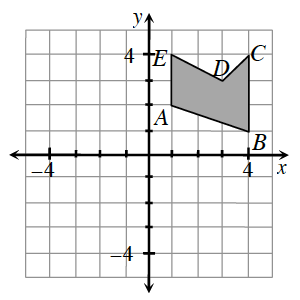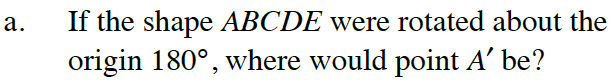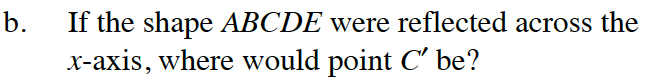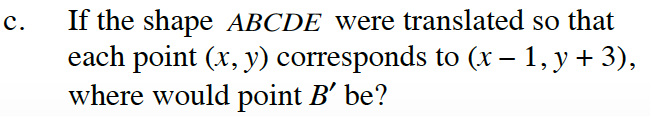Use the eTool below to help you with this problem.
Click on the link to the right to view the full version of the eTool. Int1 11-88 HW eTool

A' = (−1, −2)

Move left 1 and up 3.

C' = (4, −4)

B' = (3, 4)